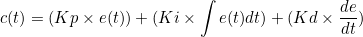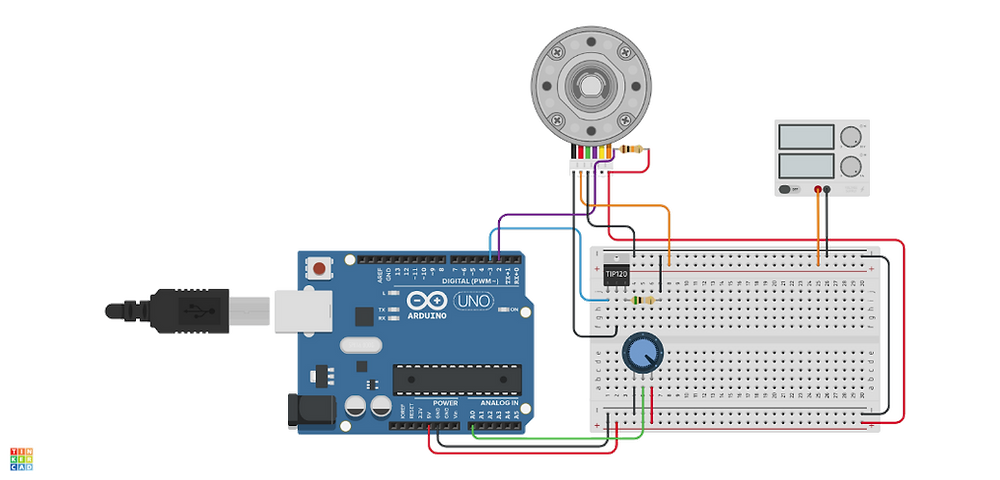•UFRJ Nautilus

# PID Control With Arduino

Updated: Jun 25, 2021

How can we command a robot so that it maintains a position and orientation under water? In this post we will see how a PID controller can be used to solve this and other problems in robotics.

There are different control algorithms that can be used in our systems, but the most common closed control algorithm is probably the PID. To understand it in an intuitive way, we first need to understand what a closed control is. Let’s think of the case of a driver in tortuous terrain. The driver wishes to keep his vehicle in the speed limit. This is the wish of the controller, and we will call it “setpoint”. The driver controls the acceleration of the car, which we will call “plant”, through the gas and brake pedals. He can also observe the changes in the car’s speed by looking at the speed meter.

This is probably the simplest and most daily example we have of a closed control system, where the driver uses the observation of the car to control it using the gas and brake pedals. The diagram for this system would be:Now that we know what a closed control system is, also called a feedback control system, we can talk about the PID, an acronym for Proportional, Integral, Derivative. This controller will use the current error (Proportional), its integral (Integral) and its derivative (Derivative) to generate an output we will use to act in our system. Its diagram would look like the following:The equation that describes the output for this diagram as a function of time is the following:We will implement this controller with the objective of controlling the speed of a DC motor using an arduino.

In order to do so, we will use the discrete version of the equation above, substituting the integral by a summation and rewriting the error variation in a discrete way.Our circuit consists of a motor we wish to control, with an encoder, a sensor capable of measuring the speed of the motor, and a potentiometer we can use to choose the speed of the motor.

Implementation of the controller:

```// Hardware Mapping
#define PWM_OUT       3
#define ENCODER_A 2
#define POT_IN A0

int output;           // defines the PWM output
int speed;            // motor speed
int lastError = 0;    // declares the last error
int setpoint;         // declares the setpoint
int error = 0;        // current error
int I_error = 0;      // integral gain
int D_error = 0;      // derivative gain
float KP = 0.1;       // proportional constant
float KI = 0.0001;    // integrative constant
float KD = 0.001;     // derivative constant

void setup() {
Serial.begin(9600);              // begins the serial communication

pinMode(ENCODER_A, INPUT);      // sets the encoder as an input
pinMode(POT_IN, INPUT);     // sets the potentiometer as an input and controller of the setpoint value
pinMode(PWM_OUT, OUTPUT);          // sets pin 3 as a PWM output for the speed control

output = 10;              // sets an initial value for PWM_OUT
analogWrite(PWM_OUT, output);   // controls the PWM value through the output in pin 3
}

void loop() {
// calculates the setpoint value from the analog reading in the potentiometer

// transforms the reading from the potentiometer into speed pulses

speed = 19.1*((60*1000*10) / pulseIn(ENCODER_A, HIGH));

error = setpoint - speed; // calculates the error value

I_error += error; // error summation
D_error = lastError - error; // error variation
// as this loop will be called regularly, we can suppose
// that dt is a constant and not include it in the above constants.
// Dt is alongside KP, KI, KD, in an implicit way.

// Implementation of the PID algorithm

output += KP*error + KI*I_error + KD*D_error;

// limits the control output to valid PWM values (10-255)
output = constrain(output, 10, 255);

// sends the signal to the motor
analogWrite(PWM_OUT, output);

// prints the speed and setpoint values
// RPM values
// use the graph funcion in the serial monitor!
Serial.print(speed);
Serial.print(",");
Serial.println(setpoint);
}

```

Note that since we wish to control the speed of a motor, when the motor’s speed is equal to the setpoint, the code will send an empty signal to the motor, making it slow down, distancing its value from the setpoint. To solve this problem, we sum the previous output of the controller to the new output, so that when the error gets close to 0, the controller will keep sending the signal that takes the motor to the desired speed.

Another modification we made in the above implementation is the exclusion of the time-related terms, because we are calling the controller in a loop with a constant time interval, approximately. That way, if dt is constant we can make the following simplifications:Not only do these simplifications save us processing cycles in our arduino, but they also make the code’s implementation easier!